Question

# A ?1 octahedral complex is found to absorb visible light, with the absorption maximum occurring at 499 nm . Calculate the crystal-field splitting energy, Δ , in kJ/mol.

A octahedral complex is found to absorb visible light, with the absorption maximum occurring at

Calculate the crystal-field splitting energy,

in kJ/mol.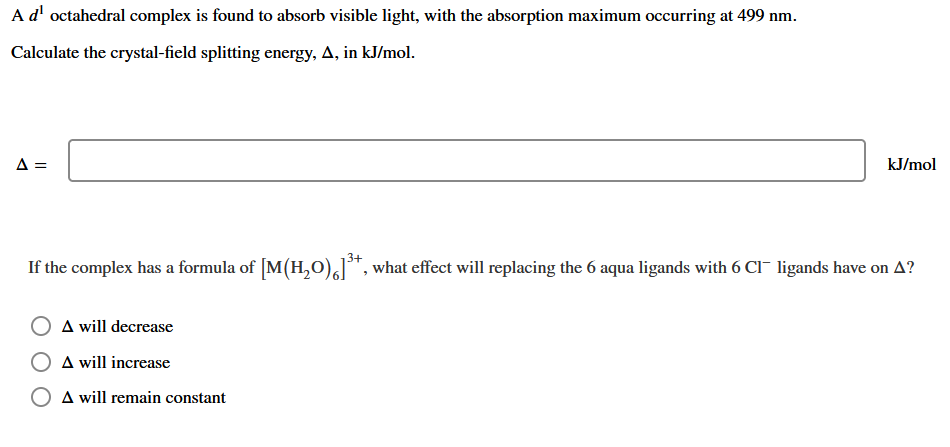We need at least 10 more requests to produce the answer.

0 / 10 have requested this problem solution

The more requests, the faster the answer.

All students who have requested the answer will be notified once they are available.

#### Earn Coins

Coins can be redeemed for fabulous gifts.

Similar Homework Help Questions
• ### A dl octahedral complex is found to absorb visible light, with the absorption maximum occurring at...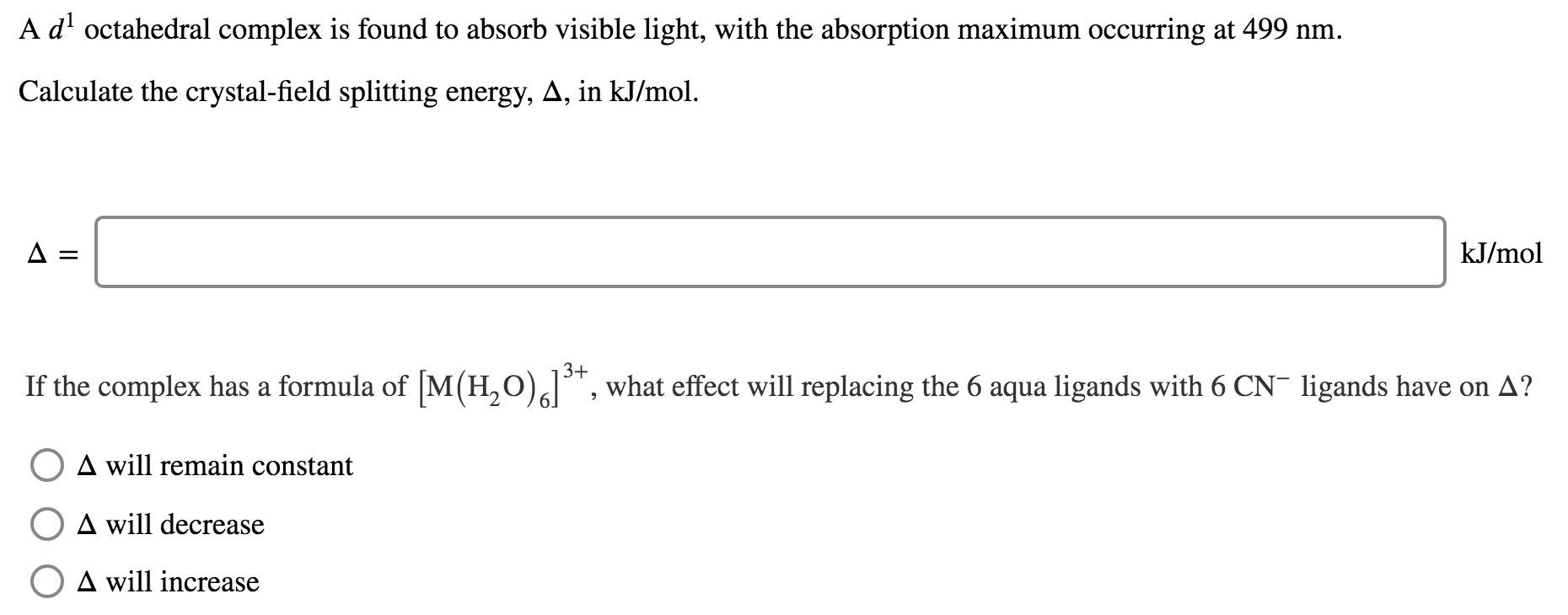A dl octahedral complex is found to absorb visible light, with the absorption maximum occurring at 499 nm. Calculate the crystal-field splitting energy, A, in kJ/mol. A= kJ/mol If the complex has a formula of [M(H20)alt, what effect will replacing the 6 aqua ligands with 6 CN- ligands have on A? O A will remain constant A will decrease O A will increase

• ### Ad octahedral complex is found to absorb visible light, with the absorption maximum occurring at 515...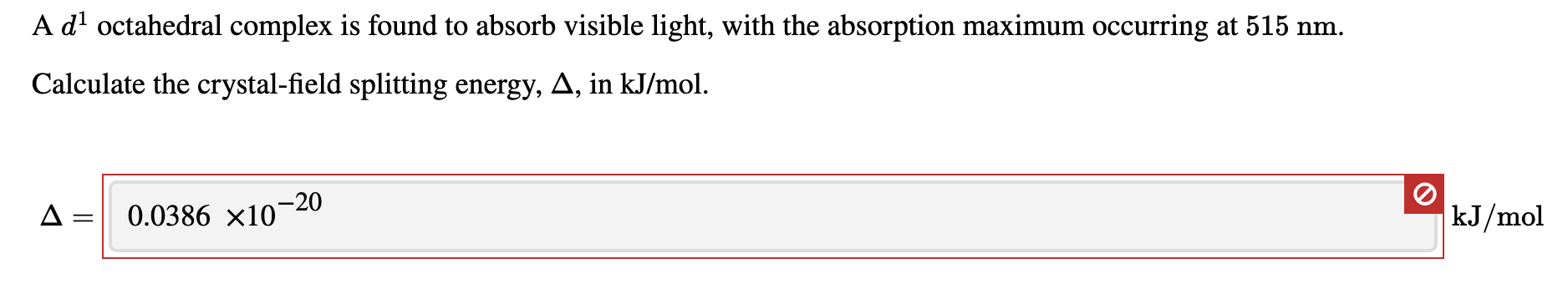Ad octahedral complex is found to absorb visible light, with the absorption maximum occurring at 515 nm. Calculate the crystal-field splitting energy, A, in kJ/mol. A = 0.0386 x10-20 kJ/mol

• ### 13. An octahedral transition metal complex with a d' electron configuration absorbs light of wavelength equal to 650 nm. a. what is the magnitude of the octahedral crystal field splitting par...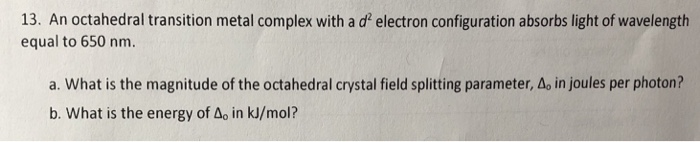13. An octahedral transition metal complex with a d' electron configuration absorbs light of wavelength equal to 650 nm. a. what is the magnitude of the octahedral crystal field splitting parameter, Δο in joules per photon? b. What is the energy of Ao in kJ/mol? 13. An octahedral transition metal complex with a d' electron configuration absorbs light of wavelength equal to 650 nm. a. what is the magnitude of the octahedral crystal field splitting parameter, Δο in joules per...

• ### An octahedral metal complex absorbs light with wavelength 580 nm. a. What is the crystal field...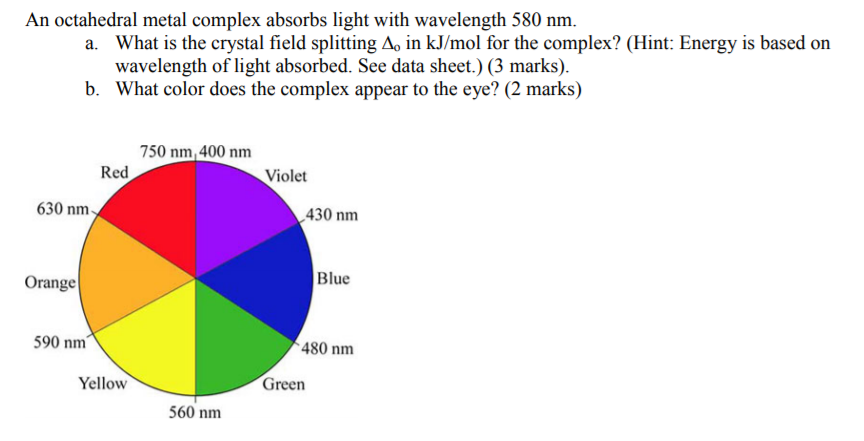An octahedral metal complex absorbs light with wavelength 580 nm. a. What is the crystal field splitting A, in kJ/mol for the complex? (Hint: Energy is based on wavelength of light absorbed. See data sheet.) (3 marks) b. What color does the complex appear to the eye? (2 marks) 750 nm, 400 nm Red Violet 630 nm 430 nm Blue Orange 590 nm 480 nm Yellow Green 560 nm

• ### Calculate the crystal field stabilization energy valiue for the complex Cr(NH)J*. The crystal field splitting energy...Calculate the crystal field stabilization energy valiue for the complex Cr(NH)J*. The crystal field splitting energy and pairing energy are found to be 150 kJ mol and 120 kJ mol respectively. 4.(c) 10 cc. Calculate the crystal field stabilization energy valiue for the complex Cr(NH)J*. The crystal field splitting energy and pairing energy are found to be 150 kJ mol and 120 kJ mol respectively. 4.(c) 10 cc.

• ### Estimate the crystal field stabilization energy for the octahedral ion hexacyanocobaltate(III), if the wavelength of maximum...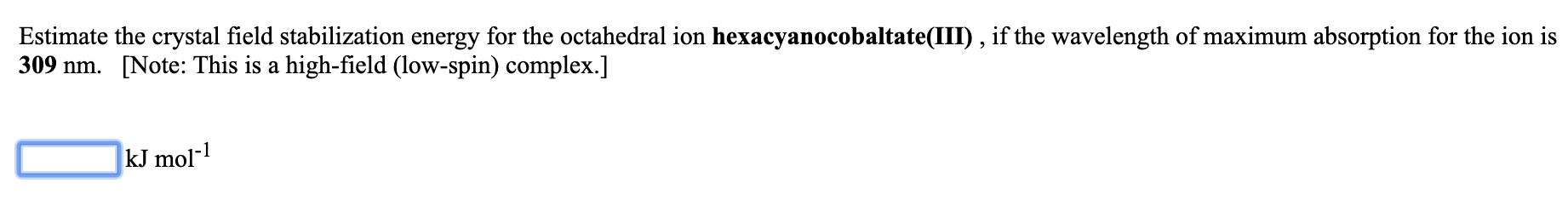Estimate the crystal field stabilization energy for the octahedral ion hexacyanocobaltate(III), if the wavelength of maximum absorption for the ion is 309 nm. [Note: This is a high-field (low-spin) complex.] kJ mol-1

• ### Estimate the crystal field stabilization energy for the octahedral ion hexafluorocobaltate(III), if the wavelength of maximum...

Estimate the crystal field stabilization energy for the octahedral ion hexafluorocobaltate(III), if the wavelength of maximum absorption for the ion is 710 nm.   [Note: This is a weak-field (high-spin) complex.] _____ kJ mol-1

• ### Estimate the crystal field stabilization energy for the octahedral ion hexacyanomanganate(III), if the wavelength of maximum...

Estimate the crystal field stabilization energy for the octahedral ion hexacyanomanganate(III), if the wavelength of maximum absorption for the ion is 600 nm. [Note: This is a high-field (low-spin) complex.] kJ mol-1

• ### 620 nm Estimate the wavelength of maximum absorption for the octahedral ion trioxalatochromate(III) from the fact...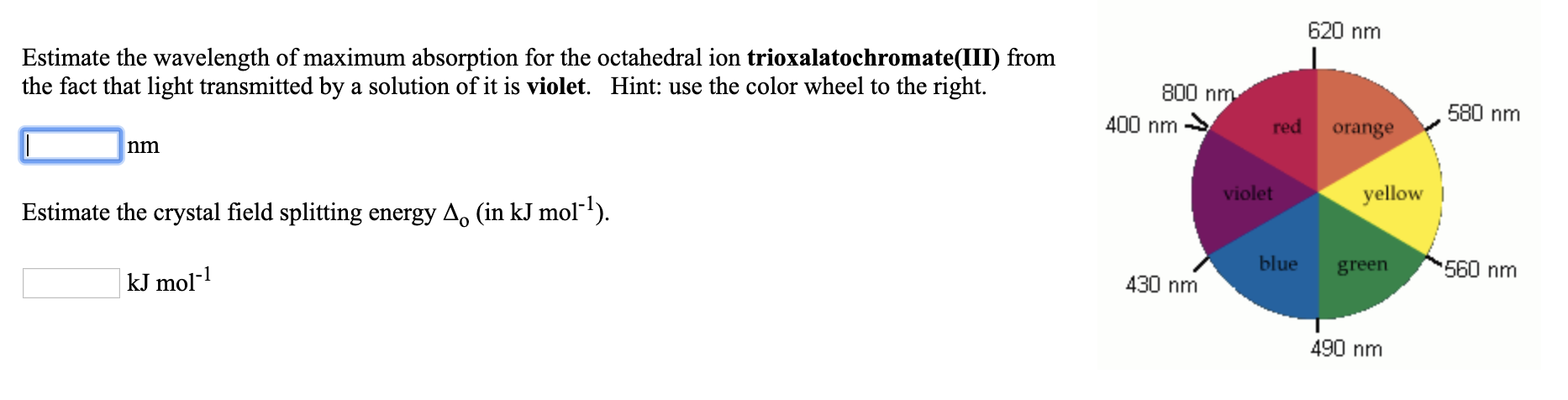620 nm Estimate the wavelength of maximum absorption for the octahedral ion trioxalatochromate(III) from the fact that light transmitted by a solution of it is violet. Hint: use the color wheel to the right. 800 nm 400 nm → red orange 580 nm violet yellow Estimate the crystal field splitting energy A. (in kJ mol-?). blue green kJ mol-1 560 nm 430 nm 490 nm

• ### The complex [Fe(H2O)6]3+ has a maximum absorbance at 724 nm. a.Calculate the crystal field splitting energy,...

The complex [Fe(H2O)6]3+ has a maximum absorbance at 724 nm. a.Calculate the crystal field splitting energy, in kJ, for the complex. b.Predict the colour of the complex.